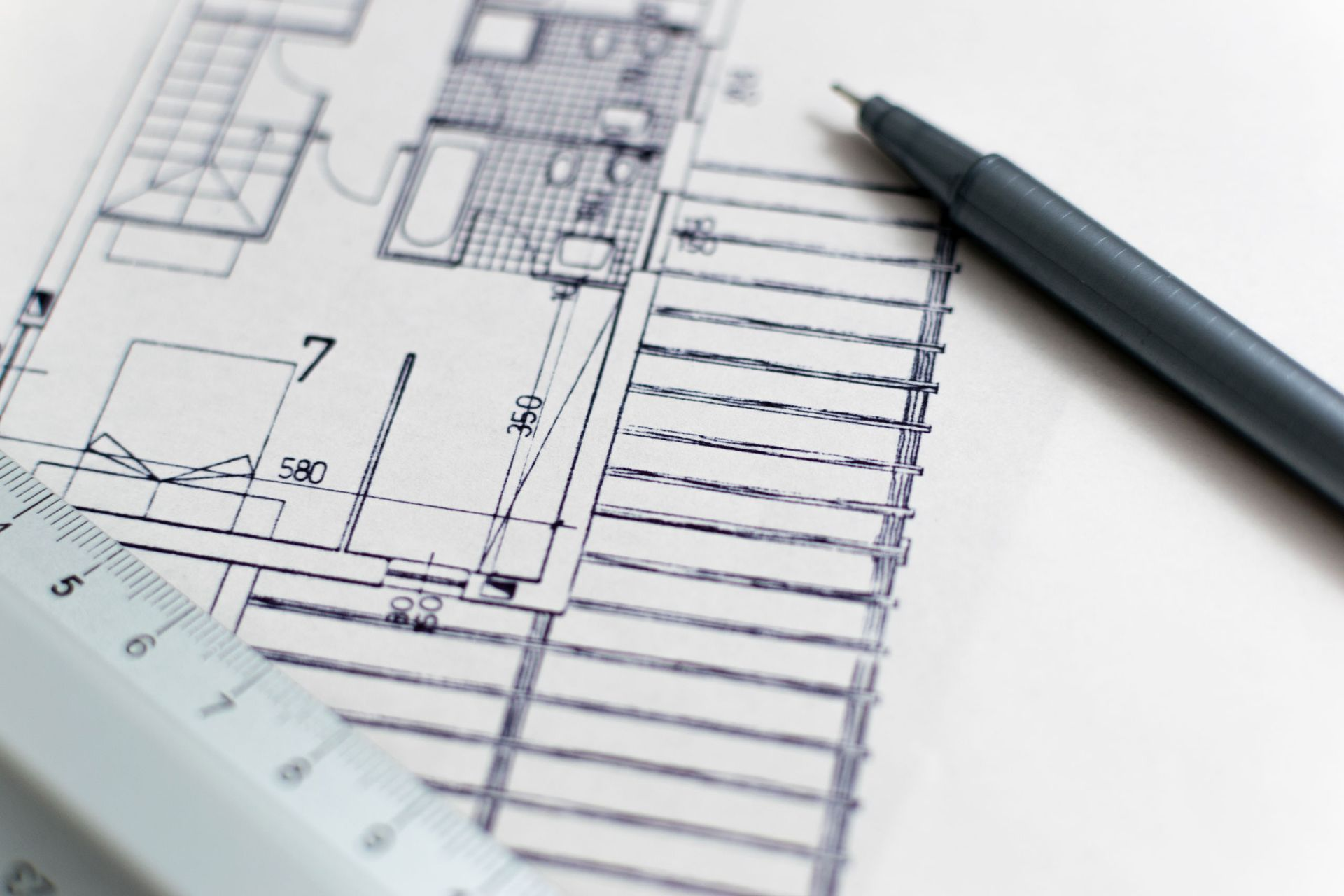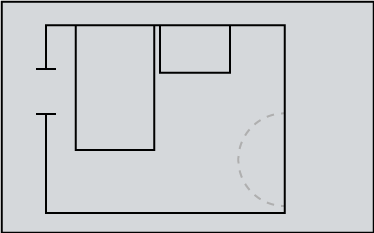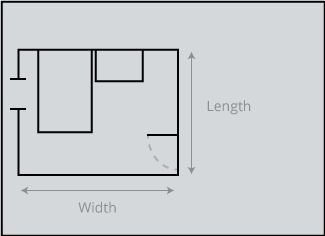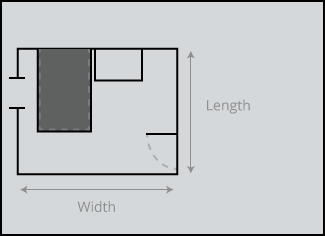Many home improvement projects require you to measure the space you will be working in. Renovations such as installing new flooring and painting walls, require such measurements to be made, for example, if you’re putting in flooring, you need to know the area of the floor and if you are painting, you need to know the area of the walls and ceiling. This can look difficult if you have never done it before. If there are built-in features like differently shaped ceilings, walls, recesses, and bay windows – there can be an added level of difficulty.

Step 1: Begin by drawing a floor plan in the room you are measuring. You can use this drawing to record your measurements and it does not have to be to scale. It will just be used as a reference. All areas involved in your project should be included. Of course, since it is a floor, windows and doorways should not matter. For example, if you’re putting in flooring and have a walk-in space for storage or a closet, you are also flooring that space. Draw it on the floor plan.Step 2: Next you need to measure the length and width of the room. Right now, you are only measuring the main area. You might need to move furniture out of the way.Step 3: To convert among square feet, square inches, square yards, square centimetres, square millimetres and square meters you can utilize the conversion table located at the bottom of our calculators. Our tools give you the option of calculating the exact cost of materials. All you have to do is enter the price per unit area and voila, you have the total cost of materials in a single click! To calculate the area of a square or rectangular room, use the standard formula on our square footage calculator. You might need a combination of the below calculators to figure out the exact area of the structure you are trying to cover:

Area of A Square
The area of a square is the space contained within a set of lines. These lines should be measured in feet (ft) for square footage calculations and if needed, converted to inches (in), yards (yd), centimetres (cm), millimetres (mm) and metres (m).

#### Formula:

Area of a Square= Side length x Side length
Answer = (Length x length) square area
Abbreviations of unit area: ft2, in2, yd2, cm2, mm2, m2

Area of a Rectangle
The area of a square is the space contained within a set of parallel lines – each set of different lengths. These lines should be measured in feet (ft) for square footage calculations and if needed, converted to inches (in), yards (yd), centimetres (cm), millimetres (mm) and metres (m).

#### Formula:

Area of a Square= Side length x Side width
Answer = (Length x Width) square area
Abbreviations of unit area: ft2, in2, yd2, cm2, mm2, m2

Area of a Triangle
The area of a triangle is the space contained within its 3 sides. To find out the area of a triangle, we need to know the length of its three sides. The sides should be measured in feet (ft) for square footage calculations and if needed, converted to inches (in), yards (yd), centimetres (cm), millimetres (mm) and metres (m).

#### Formula:

Area of a Triangle = (1/4) x √ [ (a+b+c) x (b+c-a) x (c+a-b) x (a+b-c) ]
Answer = ((1/4) x √ [ (a+b+c) x (b+c-a) x (c+a-b) x (a+b-c) ])
Abbreviations of unit area: ft2, in2, yd2, cm2, mm2, m2

Area of a Circle
The area of a circle is the space contained within its circumference (outer perimeter). To find out the area of a circle, we need to know its diameter which is the length of its widest part. The diameter should be measured in feet (ft) for square footage calculations and if needed, converted to inches (in), yards (yd), centimetres (cm), millimetres (mm) and metres (m).

#### Formula:

Area of a Circle = π x (Diameter/2)2
π = 3.142
Answer = (π x (Diameter/2)2) square area
Abbreviations of unit area: ft2, in2, yd2, cm2, mm2, m2

Area of a Trapezoid
To find out the area of a trapezoid, we need to know the length of two parallel sides and the distance (height) between them. The sides should be measured in feet (ft) for square footage calculations and if needed, converted to inches (in), yards (yd), centimetres (cm), millimetres (mm) and metres (m).

#### Formula:

Area of a Trapezoid = ((a + b) / 2) h
Answer = (((a + b) / 2) h) square area
Abbreviations of unit area: ft2, in2, yd2, cm2, mm2, m2

Step 4: If there is more than one floor area, add all of the areas together to get the total floor area. Add the areas of all recesses to the main floor area. Now, you have the total square footage of your floor, and you can purchase carpet, flooring, or other materials accordingly. Happy measuring!NOTE: Neglecting to buy extra materials for your project, will definitely leave you with a deficit, so make sure to buy extra material with a margin of 5-10% depending on the shape of your room and the type of boards. Laminate floorboards in a square room are likely to lose less in offcuts and waste than natural hardwood in an irregular shaped room.